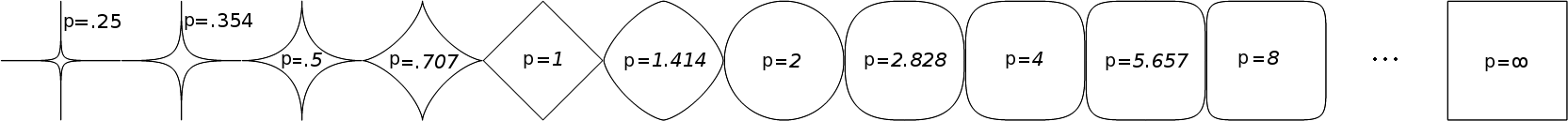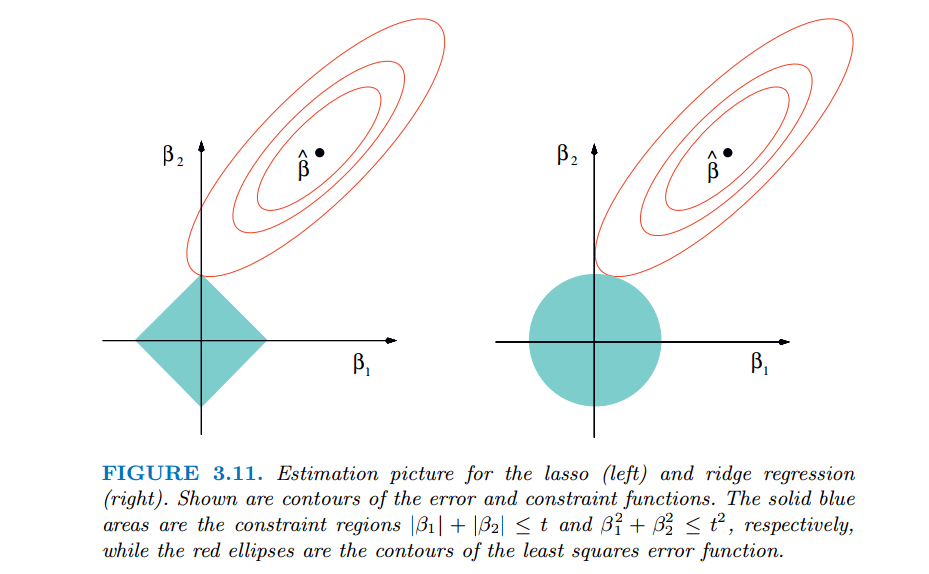0%

# 前言

1. 脏数据太多，需要清洗数据
2. 增加训练数据的数量和多样性
3. 特征数量过多，使用正则化减少特征数量

# 向量的范数

L0范数：向量中非零元素的个数。
L1范数：向量中各个元素绝对值之和，又叫“稀疏规则算子”（Lasso regularization）
L2范数：向量中各个元素平方和再开方
p-范数：$||\textbf{x}||_p = (\sum_{i=1}^N|x_i|^p)^{\frac{1}{p}}$，即向量元素绝对值的p次方和的1/p次幂。# 正则化

## Lasso 回归

Lasso 算法（英语：least absolute shrinkage and selection operator，又译最小绝对值收敛和选择算子、套索算法）是一种同时进行特征选择和正则化（数学）的回归分析方法，旨在增强统计模型的预测准确性和可解释性，最初由斯坦福大学统计学教授 Robert Tibshirani 于 1996 年基于 Leo Breiman 的非负参数推断(Nonnegative Garrote, NNG)提出。

Lasso 回归：min $1/N\ast\sum_{i = 1}^{N}{(y_{i} -\omega^{T} x_{i})^{2} } + \lambda||\omega||_{1}$

## Ridge 回归

Ridge 回归：min $1/N\ast\sum_{i = 1}^{N}{(y_{i} -\omega^{T} x_{i})^{2} } + \lambda ||\omega||_{2}^{2}$

## 比较两者

Lasso 回归与 Ridge 回归有共同点，也有区别。

### 共同点

1. 线性回归出现的过拟合现象
2. 使用 Normal equation 求解时，解决 $(X^TX)$ 不可逆的问题。

### 区别